Create a new printable
Answer key also includes questions
Answer key only gives the answers
No answer keySpring
March
Math Worksheets

Sample - Click above to make a new math worksheet (PDF).
 Name _____________________________Date ___________________
Spring
Use the clues to figure out which pictures to draw. The solution is a sequence of 4 different pictures. The order of the pictures is important.

1.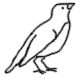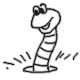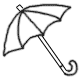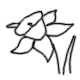1 One of the pictures in this row is in the solution.
 0 None of those pictures is in the exact position of the solution.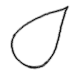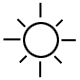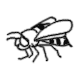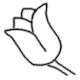1 One of the pictures in this row is in the solution.
 0 None of those pictures is in the exact position of the solution.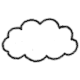1 One of the pictures in this row is in the solution.
 0 None of those pictures is in the exact position of the solution.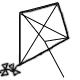1 One of the pictures in this row is in the solution.
 0 None of those pictures is in the exact position of the solution.3 Three of the pictures in this row are in the solution.
 1 One of those pictures is in the exact position of the solution.1 One of the pictures in this row is in the solution.
 0 None of those pictures is in the exact position of the solution.2 Two of the pictures in this row are in the solution.
 2 Two of those pictures are in the exact position of the solution.

Draw the solution:

______________      ______________      ______________      ______________

 Answer Key

Sample
This is only a sample worksheet.

Create a new printable
Answer key also includes questions
Answer key only gives the answers
No answer keySpring
March
Math Worksheets